## Graphs in Java: Representing Graphs in Code

### Introduction

Graphs are a convenient way to store certain types of data. The concept was ported from mathematics and appropriated for the needs of computer science.

Due to the fact that many things can be represented as graphs, graph traversal has become a common task, especially used in data science and machine learning. Graph traversal refers to the process of visiting nodes (aka vertices) in a graph via the connecting edges. This is commonly used for finding a particular node in the graph, or for mapping out a graph.

In this series we'll be taking a look at how graphs are used and represented in computer science, as well as some popular traversal algorithms:

### Representing Graphs in Code

Now that we've acquainted ourselves with what graphs are and when they're useful, we ought to know how to implement them in code.

The main two approaches to this problem are adjacency matrices and adjacency lists.

Let's start with the assumption that we have n nodes and they're conveniently named 0,1,...n-1 and that they contain the same value whose name they have. This rarely happens of course, but it makes explaining the adjacency matrix easier.

The situation where our nodes/vertices are objects (like they most likely would be) is highly complicated and requires a lot of maintenance methods that make adjacency matrices more trouble than they're worth most of the time, so we'll only provide the implementation of the "simple" case.

Let's say that we have the following graph: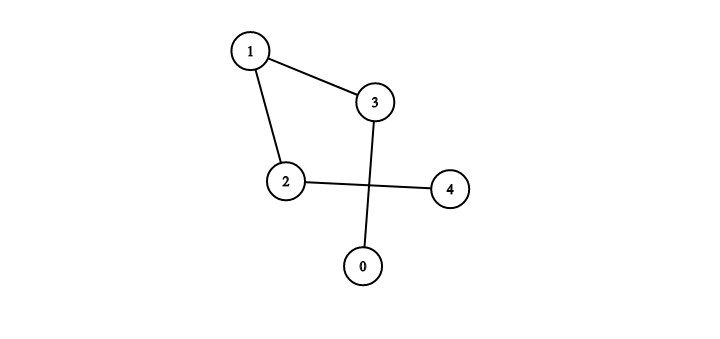In this graph, there are 5 nodes - (0,1,2,3,4) with the edges {1,2}, {1,3}, {2,4}, {3,0}. By definition, when we look at an unweighted undirected graph - the position (i,j) in our adjacency matrix is 1 if an edge exists between nodes i and j, otherwise it's 0. In the case of an undirected graph the adjacency matrix is symmetrical.

The adjacency matrix of the previous example would look like this: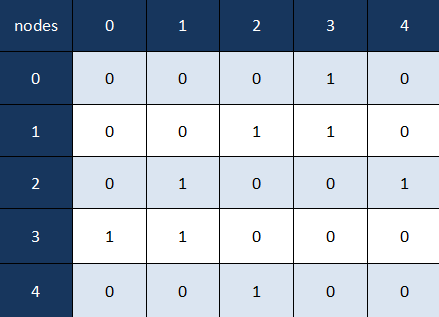We could reverse the process as well, draw a graph from a given adjacency matrix.

We'll give an example of the reverse process but with an adjacency matrix of a weighted graph. In this case the position (i,j) in our matrix is equal to the weight of the edge between nodes i and j if one exists, otherwise it is equal to infinity.

Note: Using infinity as a weight is considered a "safe" way to show that an edge doesn't exist. But, for example, if we knew that we'd only have positive weights, we could use -1 instead, or whatever suitable value we decided on.

Let's construct a weighted graph from the following adjacency matrix: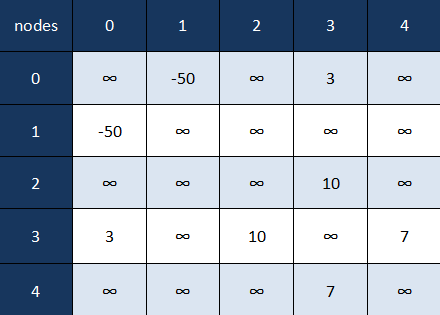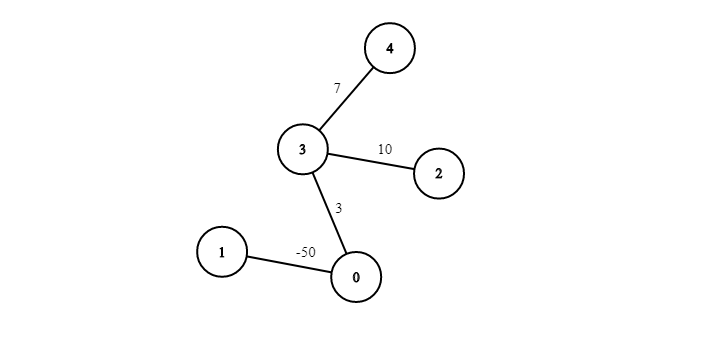As the last example we'll show how a directed weighted graph is represented with an adjacency matrix: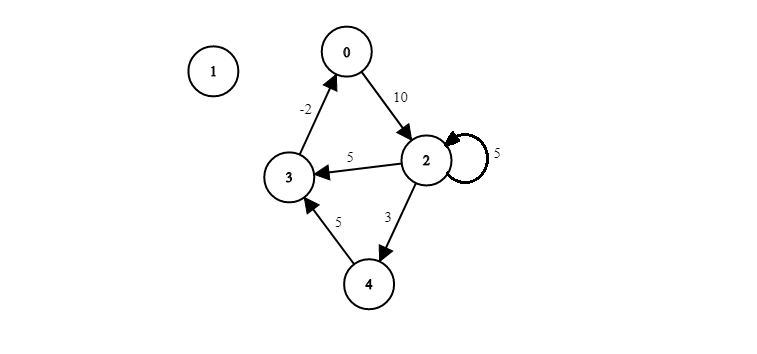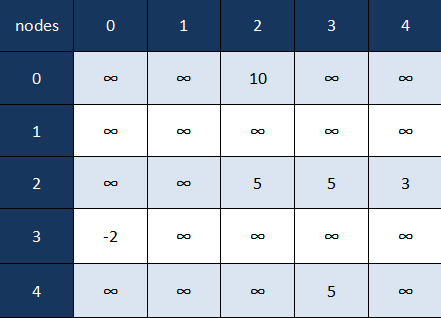Notice how with directed graphs the adjacency matrix is not symmetrical, e.g. we have a value at (0,3) but not at (3,0). Also there's no reason why a node can't be the start and end node of an edge, and we can have entirely unconnected nodes.

Now that we've seen how adjacency matrices work on paper, we need to consider their implementation. If our "nodes" were indeed simply integer values 0,1,...n-1, the implementation would be fairly straightforward.

However, since this often isn't the case, we need to figure out how we can use the convenience of using matrix indices as nodes when our nodes are objects.

In our implementation we'll be making our class as versatile as possible. This is reflected in a few more methods and some edge-cases being taken into consideration.

We'll also provide the choice between a directed and undirected graph, as well as a weighted/unweighted one.

public class Graph {

private int numOfNodes;
private boolean directed;
private boolean weighted;
private float[][] matrix;

/*
This will allow us to safely add weighted graphs in our class since
we will be able to check whether an edge exists without relying
on specific special values (like 0)
*/
private boolean[][] isSetMatrix;

// ...
}


Then, we'll have a simple constructor:

public Graph(int numOfNodes, boolean directed, boolean weighted) {

this.directed = directed;
this.weighted = weighted;
this.numOfNodes = numOfNodes;

// Simply initializes our adjacency matrix to the appropriate size
matrix = new float[numOfNodes][numOfNodes];
isSetMatrix = new boolean[numOfNodes][numOfNodes];
}


Now, let's write a method that allows us to add edges. We want to make sure that in case the graph is weighted and a weight isn't provided we set the edge value to 0, and if isn't weighted to simply add 1:

/*
Since matrices for directed graphs are symmetrical, we have to add
[destination][source] at the same time as [source][destination]
*/
public void addEdge(int source, int destination) {

if (weighted) {
}

isSetMatrix[source][destination] = true;

if (!directed) {
isSetMatrix[destination][source] = true;
}
}


In case the graph isn't weighted and a weight is provided, we simply ignore that and set the [source,destination] value to 1, indicating that an edge does exist:

public void addEdge(int source, int destination, float weight) {

if (!weighted) {
}

isSetMatrix[source][destination] = true;

if (!directed) {
isSetMatrix[destination][source] = true;
}
}


At this point, let's add a method that allows us to easily print out the adjacency matrix:

public void printMatrix() {
for (int i = 0; i < numOfNodes; i++) {
for (int j = 0; j < numOfNodes; j++) {
// We only want to print the values of those positions that have been marked as set
if (isSetMatrix[i][j])
System.out.format("%8s", String.valueOf(matrix[i][j]));
else System.out.format("%8s", "/  ");
}
System.out.println();
}
}


And after that, a convenience method that prints out the edges in a more understandable way:

/*
We look at each row, one by one.
When we're at row i, every column j that has a set value represents that an edge exists from
i to j, so we print it
*/
public void printEdges() {
for (int i = 0; i < numOfNodes; i++) {
System.out.print("Node " + i + " is connected to: ");
for (int j = 0; j < numOfNodes; j++) {
if (isSetMatrix[i][j]) {
System.out.print(j + " ");
}
}
System.out.println();
}
}


Finally, let's write two helper methods that'll be used later on:

public boolean hasEdge(int source, int destination) {
return isSetMatrix[source][destination];
}

public Float getEdgeValue(int source, int destination) {
if (!weighted || !isSetMatrix[source][destination])
return null;
return matrix[source][destination];
}


To showcase how an adjacency matrix works, let's use our class to make a graph, populate it with relations, and print them:

public class GraphShow {
public static void main(String[] args) {

// Graph(numOfNodes, directed, weighted)
Graph graph = new Graph(5, false, true);

graph.addEdge(1, 3); // The default weight is 0 if weighted == true

graph.printMatrix();

System.out.println();
System.out.println();

graph.printEdges();

System.out.println();
System.out.println("Does an edge from 1 to 0 exist?");
if (graph.hasEdge(0,1)) {
System.out.println("Yes");
}
else System.out.println("No");
}
}


Which gives us the output:

     /       /      19.0    -2.0     /
/       /       3.0     0.0     0.0
19.0     3.0     /       0.0     /
-2.0     0.0     0.0     /       0.0
/       0.0     /       0.0     /

Node 0 is connected to: 2 3
Node 1 is connected to: 2 3 4
Node 2 is connected to: 0 1 3
Node 3 is connected to: 0 1 2 4
Node 4 is connected to: 1 3

Does an edge from 1 to 0 exist?
No
null


If we constructed a graph based on this matrix, it would look like the following: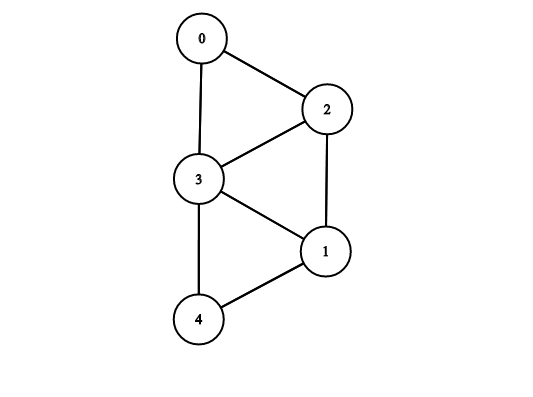Adjacency lists are much more intuitive to implement and are used a lot more often than adjacency matrices.

As the name implies, we use lists to represent all nodes that our node has an edge to. Most often this is implemented with HashMaps and LinkedLists.Adjacency lists favor directed graphs, since that is where they are most straight-forward, with undirected graphs requiring just a little more maintenance.

In this example we can see that:

Node 0 is connected with node 3
Node 1 is connected with nodes 3, 2
Node 2 is connected with nodes 1, 4
Node 3 is connected with nodes 1, 0
Node 4 is connected with node 2


It's obvious that for node 0 we would create a LinkedList that contains the node 3. For node 1 we'd create a LinkedList containing nodes 3 and 2, and so on.

For weighted graphs, like the one below, we'd need lists of arrays instead of lists of nodes. The arrays would contain the node at the other end of the edge as the first parameter, and the associated weight as the second.0: [1,-50] -> [3,3]
1: [0,-50]
2: [3, 10]
3: [0,3] -> [2,10] -> 4,7
4: [3,7]0: [2,10]
1: null
2: [2,5] -> [3,5] -> [4,3]
3: [0,-2]
4: [3,5]


One great thing about adjacency lists is that working with objects is much easier than with an adjacency matrix.

We'll be implementing adjacency lists with objects as nodes, as opposed to indexes. This is both favored when explaining adjacency lists and is more useful to know, as you'll likely work with objects in a project.

The code might seem complex at first glance but it's rather straight-forward when you look closely. First, let's start off with a simple Node class:

public class Node {
int n;
String name;

Node(int n, String name){
this.n = n;
this.name = name;
}
}


Now, let's define a Graph:

public class Graph {

// Each node maps to a list of all his neighbors
private boolean directed;

public Graph(boolean directed) {
this.directed = directed;
}


Now, let's add the method addEdge(). Although this time around we'll use two methods, a helper method and the actual method.

In the helper method, we'll also make a check for possible duplicate edges. Before adding an edge between A and B, we'll first remove it and only then add it. If it existed (we're adding a duplicate edge), it was removed and after adding it again, there's only one.

Though, if it didn't exist, removing a non-existing edge will result in a NullPointerException so we're introducing a temporary copy of the list:

public void addEdgeHelper(Node a, Node b) {

if (tmp != null) {
tmp.remove(b);
}
}

public void addEdge(Node source, Node destination) {

// We make sure that every used node shows up in our .keySet()

// If a graph is undirected, we want to add an edge from destination to source as well
if (!directed) {
}
}


Finally, we'll have the printEdges() and hasEdge() helper methods, which are pretty straightforward:

public void printEdges() {
for (Node node : adjacencyMap.keySet()) {
System.out.print("The " + node.name + " has an edge towards: ");
for (Node neighbor : adjacencyMap.get(node)) {
System.out.print(neighbor.name + " ");
}
System.out.println();
}
else {
System.out.println("none");
}
}
}

public boolean hasEdge(Node source, Node destination) {
}


To showcase how adjacency lists work, let's instantiate several nodes and populate a graph with them:

public class GraphShow {
public static void main(String[] args) {

Graph graph = new Graph(true);
Node a = new Node(0, "A");
Node b = new Node(1, "B");
Node c = new Node(2, "C");
Node d = new Node(3, "D");
Node e = new Node(4, "E");

graph.printEdges();

System.out.println(graph.hasEdge(a,b));
System.out.println(graph.hasEdge(d,a));
}
}


We get the output:

The A has an edge towards: B
The B has an edge towards: C D A
The C has an edge towards: E
true
false


Note: This of course heavily depends on how Java treats objects in memory. We have to make sure that further changes to our a node in main, after we have added it to our graph, will reflect on our graph! Sometimes this is what we aim for, but sometimes it isn't. Either way, we should be aware that in this case, the a node in our graph is the same as the a node in main.

We could have implemented this differently of course. Another popular approach is to add the list of outgoing edges to the Node object itself and change the Graph class appropriately:

public class Node {
int n;
String name;

Node(int n, String name) {
this.n = n;
this.name = name;
}

}
}


Both approaches are in the spirit of the Object-Oriented encapsulation concept in their own way, so either is fine.

Adjacency matrices have a much faster look-up time than adjacency lists. For example, if we wanted to check whether node 0 has an edge leading to node 4 we could just check the matrix at indices [0,4] which gives us constant execution time.

On the other hand, we would potentially need to check the whole list of 0's neighbors in its adjacency list to find whether there's an edge leading to node 4, which gives us linear (O(n)) look-up time.

Adding edges is also much faster in adjacency matrices - simply change the value at position [i,j] to add an edge from node i to node j, while with lists (if we don't have access to the pointer to the last element) can also take O(n) time, especially if we need to check whether that edge already exists in the list or not.

As far as space is concerned - adjacency lists are much more efficient, for a very simple reason. Most real-life graphs are what we call sparse, meaning that there are much fewer edges than the maximum number of edges possible.

Why is this important? Well, in an adjacency matrix we always have an n x n sized matrix (where n is the number of nodes), regardless of whether we have only a few edges or almost the maximum number (where every node is connected to every other).

In reality, this takes up a lot of space that isn't necessary, since as we said, most real-life graphs are sparse, and most of those edges we've allotted space to don't exist. Adjacency lists on the other hand only keep track of existing edges.

In more concrete terms, if we had a graph with N nodes and E edges, the space complexity of these two approaches would be: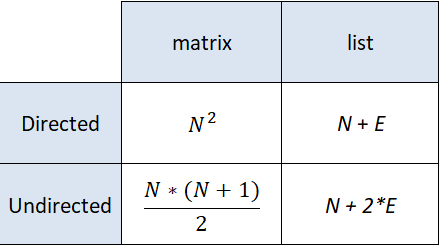#### Which Should I Choose to Implement?

Short answer - adjacency lists. They're more straight-forward when working with objects, and most of the time we don't care about the slightly better look-up time that adjacency matrices provide when compares to code maintenance and readability.

However, if we're dealing with a highly dense (opposite of sparse) graph, it could be worthwhile to invest the necessary memory to implement our graph via an adjacency matrix.

So, for example, if the operation you're most likely going to use is:

• Checking whether an edge is part of a graph: adjacency matrix, since checking whether an edge is part of a graph takes O(1) time, while in adjacency lists it takes O(lengthOfList) time
• Adding or removing edges from the graph: adjacency matrix, same difference as in the previous case
• Traversing the graph: adjacency list, takes O(N + E) time instead of O(N^2)

### Conclusion

Graphs are a convenient way to store certain types of data. The concept was ported from mathematics and appropriated for the needs of computer science.

Due to the fact that many things can be represented as graphs, graph traversal has become a common task, especially used in data science and machine learning.

The main two approaches to representing graphs in code are adjacency matrices and adjacency lists.Hey guys, I want to point out that I don't have any social media to avoid mistakes. If you need any help - post it in the comments :)